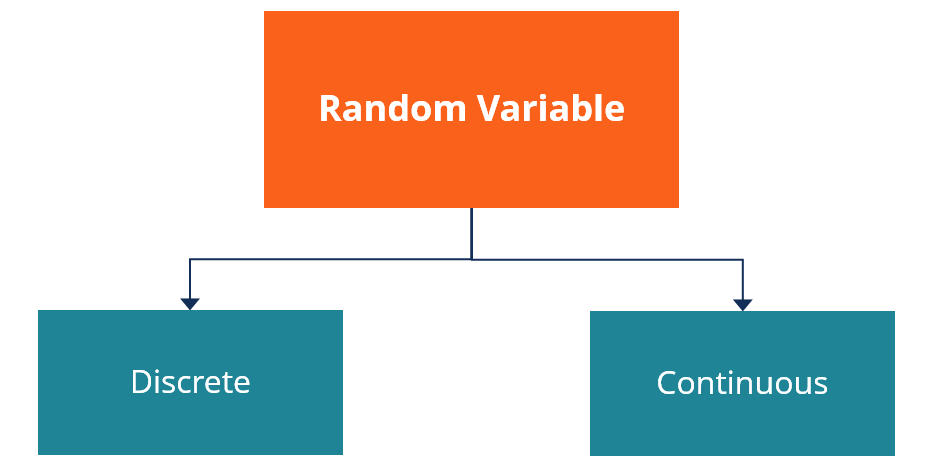# Random Variable

A type of variable in statistics whose possible values depend on the outcomes of a certain random phenomenon

## What is a Random Variable?

A random variable (stochastic variable) is a type of variable in statistics whose possible values depend on the outcomes of a certain random phenomenon. Since a random variable can take on different values, it is commonly labeled as letters (e.g., variable “X”). Each variable possesses a specific probability distribution function (a mathematical function that represents the probabilities of occurrence of all possible outcomes).### Types of Random Variables

Random variables are classified into discrete and continuous variables. The main difference between the two categories is the type of possible values that each variable can take. In addition, the type of (random) variable implies the particular method of finding a probability distribution function.

### 1. Discrete

A discrete random variable is a (random) variable whose values take only a finite number of values. The best example of a discrete variable is a dice. Throwing a dice is a purely random event. At the same time, the dice can take only a finite number of outcomes {1, 2, 3, 4, 5, and 6}.

Each outcome of a discrete random variable contains a certain probability. For example, the probability of each dice outcome is 1/6 because the outcomes are of equal probabilities. Note that the total probability outcome of a discrete variable is equal to 1.

#### 2. Continuous

Unlike discrete variables, continuous random variables can take on an uncountable infinite number of possible values. One of the examples of a continuous variable is the returns of stocks. The returns can take an infinite number of possible values (as percentages).

Due to the above reason, the probability of a certain outcome for the continuous random variable is zero. However, there is always a non-negative probability that a certain outcome will lie within the interval between two values.

### Random Variables in Finance

In finance, random variables are widely used in financial modeling, scenario analysis, and risk management. In financial models and simulations, the probabilities of the variables represent the probabilities of random phenomena that affect the price of a security or determine the risk level of an investment. For instance, a variable may be applied to indicate the price of an asset at some point in the future or signal the occurrence of an adverse event.

### More Resources

CFI is the official provider of the global Financial Modeling & Valuation Analyst (FMVA)™ certification program, designed to help anyone become a world-class financial analyst. To keep advancing your career, the additional resources below will be useful:

• Correlation
• Independent Variable
• Investing: A Beginner’s Guide
• Quantitative Analysis

### Financial Analyst Certification

Become a certified Financial Modeling and Valuation Analyst (FMVA)® by completing CFI’s online financial modeling classes and training program!